# Triangles

Print Rate 0 stars Common Core
Lesson size:
Message preview:
Someone you know has shared lesson with you:

To play this lesson, click on the link below:

https://www.turtlediary.com/lesson/triangles.html

To know more about different lessons, please visit www.turtlediary.com

Hope you have a good experience with this site and recommend to your friends too.

Login to rate activities and track progress.
Login to rate activities and track progress.

## Classification of Triangles by Angles

Triangles are used every single day, in furniture arrangement, movements, construction, and so much more.When you rest a staircase against a wall, you are making a right triangle because the wall makes a right angle with the ground, and the staircase closes the triangle.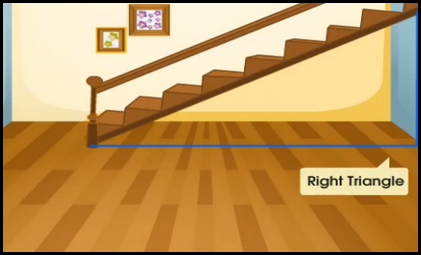When you take a shortcut across a patch of grass to get from one side of the sidewalk to the other, you are walking in a triangle. Try to notice all the ways in which you are making triangles in everyday life.Triangles have three sides and three angles - tri - (three) - angle (angles). The sum of all the angles in any triangle is 180°. Triangles are characterized by the angles within them.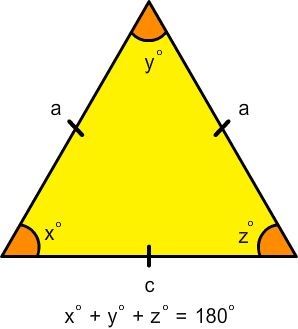There are three different types of triangles:

• Equilateral
• Isosceles
• Scalene

Lets study them in detail.

## Equilateral Triangles

The name of this triangle helps to remember its properties: Equi means equal, and lateral means sides.

Equilateral triangles have three identical sides and identical angles.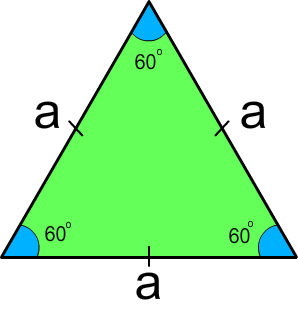No matter what is the length of the sides, equilateral triangles always have three 60° angles.

## Isosceles Triangles

Isosceles triangles have :

• Two equal sides
• Two equal angles

Two sides of a isosceles triangle are equal which means that two of its angles will also be equal.The base of a isosceles triangle is always shorter in length than the sides.

If the isosceles triangle has a right angle, its called a right isosceles triangle.

A right isosceles triangle has a right angle (90°), and two 45° angles. Right angles are shown by a square at the line intersection, rather than a curve.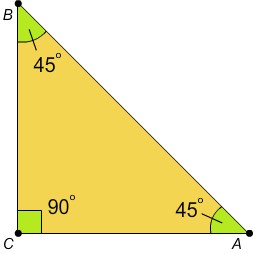## Scalene Triangles

Scalene triangles have:

• No equal sides
• No equal angles.

All of the sides and angles of a scalene triangle are different.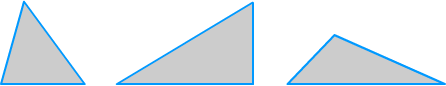Note: In scalene triangle, the Shortest side is opposite to the smallest angle and the Longest side is opposite the largest angle.

## Triangles

• Triangles have three sides and three angles - tri - (three) - angle (angles).
• The sum of all the angles in any triangle is 180°.
• There are three different types of triangles:
• Equilateral
• Isosceles
• Scalene
• Equilateral triangles have three identical sides and identical angles.
• Isosceles triangle have two side and two angles equal.
• If the isosceles triangle has a right angle, its called a right isosceles triangle.
• All of the sides and angles of a scalene triangle are different.
• Scalene Triangle has all the sides and angles different.

## Similar Lessons

Become premium member to get unlimited access.• •  Unlimited access to over thousands of worksheets and activities for all grade levels.
• •  Award-winning educational games and videos.
• •  Teacher created quizzes with step by step solution.
• •  Ad-free experience for children.
• •  Unlimited access to Interactive Stories with "Read to me" feature.
• •  Informative assessment tools with detailed reports pointing out successes and weak spots.
• •  Audio Instructions for all games.